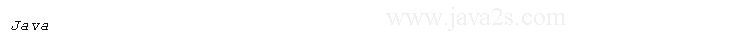# Java Reflection constructor instantiate objects

## Introduction

Through reflection, we can even create instances.

The simplest way to instantiate objects is to use `newInstance()` method of Class.

```public T newInstance()
```

This uses default constructor to instantiate objects.

The following creates an instance of String:

```String s = String.class.newInstance();
```

If we call `newInstance()` on a class that has no default constructor, an InstantiationException will be thrown.

To call constructors with parameter:

• first we find the required constructor from the class
• then call it with the right arguments.

We use `newInstance()` method of Constructor that looks like:

```public T newInstance(Object... initargs)
```

The following program illustrates how to use `newInstance()` method.

```import java.lang.reflect.Constructor;

public class Main {
public static void main(String args[]) throws Exception {
String s1 = (String) String.class.newInstance();
Class c = String.class;
Constructor con = c.getDeclaredConstructor(char[].class);
char[] chars = { 'J', 'a', 'v', 'a' };
Object[] param = { chars };
String s2 = (String) con.newInstance(param);
System.out.println(s2);/*from  www  . jav a 2 s.  com*/
}
}
```This creates two instances of String.

The first string s1 is constructed using `newInstance()` of Class.

The second string s2 is constructed with the help of the following constructor:

```public String(char[] value)
```

The `newInstance()` will throw exceptions if proper arguments are not supplied.